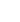10min:
LASER PRODUCED SPECTRA OF SiN MOLECLE IN BLUE -- RED REGION.

R. GOPAL, K. S. OJHA AND S. C. SINGH, Laser and Spectroscopy Lab., Department of Physics, University of Allahabad, Allahabad --211002, INDIA \noindent e - mail: spectra2@rediffmail.com.

Spectrum of SiN molecule has been observed for the first time in the region of 695 - 470 nm using laser ablation technique. Third harmonics 355nm of Nd:YAG laser (Spectra Physics, USA) was focused on the rotating target of silicon nitride (purity 99.999% Goodfellow, U.K. ) inside the ablation chamber with argon ambient atmosphere at a pressure of 10-1 torr . The produced plasma was allowed to cool adiabatically for a delay time of about 220 nsec. The radiation from this cooled plasma was focused on the slit of computer controlled Spex TRIAX 320M monochromator fitted with TE cooled ICCD. The ICCD signals were sent to the computer through SpectraMax software.

About ninety five new bands have been recorded and analyzed into three new band systems F -- X, G1 -- X and G2 -- X with (0,0) lying at about 690 nm, 551 nm and 548 nm respectively. Nineteen bands reported by Schofield and Broidaa in green region have been fitted in the G - X system. The vibrational constants of these systems are determined. The vibrational constants are as follows;

\beginarrayrllllllllll

\noindent F-X &&e=14550.93cm-1 &&e'=1030.0 &&e'xe'=2.50 &&e'ye'=0.19 &&

\noindent G1-X &&e=18262.39cm-1&&e'=925.0&&e' xe'=8.00 &&e'ye'= 0.17 &&

\noindent G2-X &&e=18384.26cm-1 &&e'=900.5&&e' xe'=4.50 &&e'ye'=0.009 &&

\noindent X&& &&e''=1151.36 &&e''xe''=6.47 &&e''ye''=0.002 &&
\endarray \_\_\_\_\_\_\_\_\_\_\_\_\_\_\_\_\_\_\_\_\_\_\_\_\_\_\_\_\_\_\_\_\_\_\_\_\_\_\_\_\_\_\_\_\_\_\_\_\_\_\_\_\_\_\_

a K. Schoffield and H. P. Broida, Photochem. Photobiol. \underline \textbf4, 989 (1965)

\textbf\_\_\_\_\_\_\_\_\_\_\_\_\_\_\_\_\_\_\_\_\_\_\_\_\_\_\_\_\_\_\_\_\_\_\_\_\_\_\_\_\_\_\_\_\_\_\_\_\_\_\_\_\_\_\_\_\_\_\_\_\_\_\_\_\_\_\_\_\_\_\_\_\_\_\_\_\_\_\_\_\_\_\_\_\_\_\_\_\_\_\_\_\_\_\_\_\_\_\_\_\_\_\_\_\_\_\_\_\_\_\_\_\_\_\_\_\_\_\_\_\_\_\_\_\_\_\_\_\_\_\_\_\_\_\_\_\_\_\_\_\_\_\_\_\_\_\_\_\_\_\_\_\_\_\_\_\_\_\_\_\_\_\_\_\_\_\_\_\_\_\_\_\_\_\_\_\_\_\_\_\_\_\_\_\_\_\_\_\_